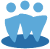## Section 5 of the SSG in Toolkit 6

This FAQ covers a handful of questions regarding Total Return Analysis (section 5) on the SSG form in Toolkit 6.

5 A: Present Full Year's Dividend - This can also be called the “indicated current yield,” and comes from the “Common Dividends(\$)” field in the Basic Data section. That figure is calculated by taking the latest quarterly dividend and multiplying it by 4.
For more on this, please see our FAQ page at:
https://www.iclub.com/faq/Home/Article?id=677

Average % payout in Section 5 B: By default, this is the average of the % Payout Column in section 3 of the SSG.
(The figures in the % payout column are calculated as Dividend divided by EPS.)
When divided by Forecast High or Forecast Average P/E, the Average Yield is the result.

The average yield is then added to the Annual Appreciation to give Compound Annual and Projected Average Total Return figures.This leads to the next question, "What is Annual Appreciation, and how is it calculated?"

Appreciation in 5 C and D: The short answer is that it’s the compounded annual growth rate (CAGR) of the share price.
This leads to the question, "What is CAGR?". According to Investopedia: "CAGR is the year-over-year growth rate of an investment over a specified period of time."
For a visual representation of the formula, and a good example of the formula in action, we suggest to visit the Investopedia page on this topic at:
https://www.investopedia.com/terms/c/cagr.asp#ixzz1j4y7vI1E

To bring this back to a short answer:
The Annual Appreciation in 5c is (roughly) the growth rate to get from the current Price to the Forecast High Price.
5d is a little more complicated, but it tries to show the average growth rate of price over time. It does this using the Selected High and low P/E, as well as the Estimated High EPS.